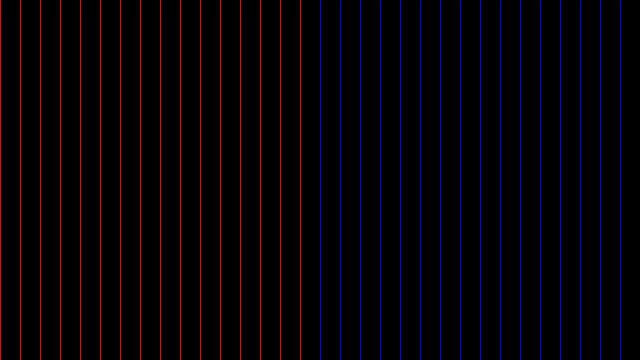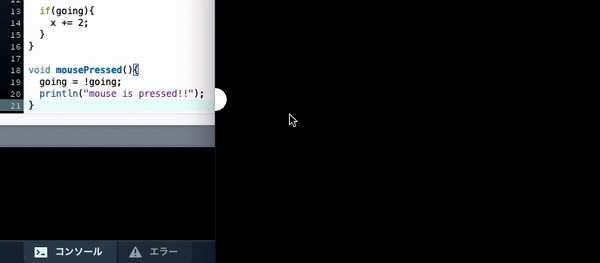# [Processing x Java] Data type and object-oriented programming

1. Object-oriented programming

# 0. Data type

## 0-0. ** What is data ...? **

Before writing about data types, we talk about "what is data". The data is information. Weight, height, eye color, car number, daylight hours, precipitation, address, images, etc. .. In programming terms, variables are data, functions are data, and classes are data.

** Point **: Data is represented in computer memory as a set of ** bits (0 and 1) **. ** Point : Data has a type ( data type **) and is saved by type.

## 0-1. ** About data types **

There are two types of data (data types): ** basic data type ** and ** composite data type **.

### ** [Basic data type] **

The base data type stores ** "only one data element" **.

name Data element size(bit) Range of values
boolean Boolean value 1 true or false
byte Part-Time Job 8 -128 to 127
char 1 alphanumeric character, symbol 16 0 to 65535
int integer 32 -2,147,483,648 to 2,147,483,647
float Floating point number 32 3.40282347E +From 38-3.40282347E + 38
color color 32 16,777,216 colors

### ** [Composite data type] **

Composite data types contain ** "multiple data and functions (methods)" **.

• Composite data types are also called ** classes **.
name Data element Method
String String length(),charAt(),equals(),indexOf(),toLowerCase(),toUpperCase()...
PFont font list()
PShape Shape beginShape(),endShape(),translate(),rotate(),scale()...
:
• Class names start with uppercase letters, so they can be easily distinguished from basic data types.

## 0-2. ** Example of using data type **

[Basic data type]

### Data type: boolean

#### `boolean01.java`

``````
//Variable declaration and initialization
boolean b = false;

size(640, 360);
background(0);
//Build-in variable width is size()It can be used only after it is defined in.
int middle = width/2;

//Loop to draw a line
for (int i = 0; i <= width; i += 20) {
//Decide when it is true and when it is false.
if (i < middle) {
b = true;
} else {
b = false;
}
//If true
if (b == true) {
stroke(255,0,0);
}
//If false
if (b == false) {
stroke(0,0,255);
}
//Draw a line
line(i,0,i,height);
}
``````#### `boolean02.java`

``````
//Define a float type variable x that indicates the x coordinate of the circle.
float x = 0;
//Define a boolean type variable going that determines whether or not the circle advances.
boolean going = false;

void setup(){
size(500,500);
}
//infinite loop(Make an animation)
void draw(){
background(0);
fill(255);
//Coordinates of the center of the circle= (x,height/2)
ellipse(x,height/2,30,30);

//If going=If True
if(going){
x += 2;
}
}

//Runs only once when the mouse is pressed
void mousePressed(){
//Substitute the truth value opposite to the current truth value for going.
//If going is True, substitute False.
//If going is False, substitute True.
going = !going;
//Outputs to the console when the mouse is pressed.
println("mouse is pressed!!");
}
``````### Data type: char

#### `char.java`

``````
//Create a char type variable letter and make initial settings.
char letter = 'A';

//char type is a character(Alphabet)Since it has the order of, use it.
for(int i = 0;i < 26;i++){
print(letter);
letter++;
}
``````
``````ABCDEFGHIJKLMNOPQRSTUVWXYZ
``````

### Data type: int

#### `int.java`

``````
//Declare a variable n of type int and initialize it.
int n = 3;

//Repeat the loop 5 times
for(int i = 0;i < 5;i++){
println(n);
// n = 2n
n *= 2;
}
``````
``````3
6
12
24
48
``````

### Data type: color

#### `color.java`

``````
//color variable blue of type color()Define using.
color blue = color(0,0,255);
background(blue);
``````[Composite data type] ----------------------------------------------------------- --------------------

### Data type: String

#### `String.java`

``````
//Create an object s1 of class String and put its contents.
String s1 = "breakfast"
//Dot(.)Use to access the methods of the object.
println(s1.length());
``````
``````9
``````

s1 is a ** String class object **, which is a ** instance variable ** in terms of variable type.

### Data type: PImage

#### `PImage.java`

``````
//Declaration of object
//Create an object called img by embodying the PImage class.
PImage img;

void setup(){
size(100,100);
//Put the contents in the object.
}

void draw(){
//Use an object.
image(img,0,0);
}
``````

# 1. Object-oriented programming

## 1-0. ** First check the terms **

** Object-oriented programming ** A program that defines related functions and variables together.

class A group of related fields and methods. It is often compared to a recipe.

field A variable defined in the class.

** Method ** A function defined in a class.

object An instance of a class (a concrete example). It has the same field names and methods as the original class. It is often compared to a dish made using a recipe.

instance An object of a class.

** Instance variable ** A type of variable. A variable created when the object was created.

variable An element of data with a certain name. Every variable has a value, a data type, and a scope.

scope The range (block) in which a variable can be accessed in a program. The place where you can use it depends on where you define it.

## 1-1. ** Programming with classes **

### ** ◯ I want to use a class **

The class is just a template and cannot be used as is. So

** "Create an object by embodying a class and treat it like a variable while taking advantage of the characteristics of the object" **

I will do it like that.

### ** ◯ Materialize the class **

The class contains ** fields and methods **. I want to use this in a program. However, the class is ** just a template ** and is an abstract entity. So, let's make the class a concrete existence (object) and operate on it.

### ** ◯ How to materialize the class **

Let's look at a simple example. Here, the object s1 is created from the class String.

#### `String.java`

``````
//Declaration of object
String s1;
//Object definition
s1 = "breakfast"
//Use of objects
println(s1.length());
``````
``````9
``````

Where s1 is ** Object ** (concrete entity made from class) And ** Instance variable ** (variable generated when an instance is created) is.

The name (appearance) differs depending on where you look from and where you set the standard, but the contents are the same. ** There are various names. ** **

### ** ◯ Treat the object like a variable while taking advantage of its characteristics **

Every object has the fields and methods that the class has.

· Field = Variable defined in the class

-Method = function defined in the class

Now that I've created the object, I think I can finally use the fields and methods of the class.

To access the fields and methods of an object, use dots (.). The object itself is treated like a variable.

#### `String.java`

``````
//Object definition
String str1 = "goodjob";
//Access the methods of the object
str1 = str1.toUpperCase();
//Use an object
println(str1);
``````
``````GOODJOB
``````

To use it like a variable ** Definition (declaration-> initial setting)-> use ** It means to use it like this.

So in summary, str1 here is

□ Has fields and methods. (Characteristics of the object) □ Use dots to access your fields and methods. (How to use) And □ Has value, data type, and scope. (Variable characteristics) □ Use after defining (declaring, initial setting). (How to use)

These are some of the ways to program with classes.

# Finally

Thank you for reading. We appreciate your opinions and suggestions in order to make the article even better.